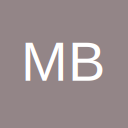# Mathematical algorithm to get an inverse of an RBG color

• Hi all,

I'm trying to create a thematic map which can adjust for negative values as well as all positive values. For instance, positive, would be lighter shades of blue to darker shades. For the values that can be negative, such as a change/delta, then I would like to incorporate red shades.

So lets say my darkest shade of blue is RGB(0,0,255) and for each lighter shade I simply up the R and G by a constant. Now what I want to do is invert it so that I can get the same intensity, so to speak, but on the other end of the spectrum. There must be a simple mathematical conversion, but I'm scratching my head with this one. Any help would be much appreciated!

• Re: Mathematical algorithm to get an inverse of an RBG color

If you can supply a few specific examples of what you mean with expected results, someone may be able to assist. For example, what would you expect to be returned based on your example of RGB(0,0,255)? Would it be RGB(255,0,0) or something else? Please try to explain the logic, as that will determine the formula required.

Is this the sort of thing you are looking for?
http://pinetools.com/invert-color

AliEnthusiastic self-taught user of MS Excel who's always learning!
If anyone has helped you towards solving a problem, then you can thank them by clicking on the like icon bottom right.• Re: Mathematical algorithm to get an inverse of an RBG color

Sure.

If you check my attached spreadsheet, I have created 3 shades of colors and supplied their respective RGB and fill codes.

How do I convert the decimal form of one blue to it's corresponding red? I feel like there should be a constant but there is no constant difference as this is a little more complex.

forum.ozgrid.com/index.php?attachment/73257/

I also believe the link you sent me is what I want. Do you know the formula to invert?

## Files

• Re: Mathematical algorithm to get an inverse of an RBG color

Sorry - can't help you with that as I don't know the algorithm. If you can find that out, then someone can create a formula for you.

AliEnthusiastic self-taught user of MS Excel who's always learning!
If anyone has helped you towards solving a problem, then you can thank them by clicking on the like icon bottom right.• Re: Mathematical algorithm to get an inverse of an RBG color

Why not use something like:

Code
``[COLOR=#666666][FONT=&quot]Range("A1").Interior.Color = RGB(255, 0, 0)[/FONT][/COLOR]``

where the color code could vary depending on the value of cell's content.
Conditional formatting would work and a vba could also do it.

• Re: Mathematical algorithm to get an inverse of an RBG color

Quote from bob22;796814

Why not use something like:

Code
``[COLOR=#666666][FONT=&quot]Range("A1").Interior.Color = RGB(255, 0, 0)[/FONT][/COLOR]``

where the color code could vary depending on the value of cell's content.
Conditional formatting would work and a vba could also do it.

I'm trying to create a heat map that responds to negative values by turning red, yet still keeping the same magnitude (i.e shade).

There is an excellent map chart tool, but it is not functioning on other versions of excel. Therefore, I'm trying to build something similar from ground up. I did come up with a solution though. PM me if you are interested.

## Participate now!

Don’t have an account yet? Register yourself now and be a part of our community!